# The SURVEYMEANS Procedure

### Computational Resources

Due to the complex nature of survey data analysis, the SURVEYMEANS procedure usually requires more memory than an analysis by the MEANS procedure for the same analysis variables. PROC SURVEYMEANS requires memory resources to keep a a copy of each unique value of the STRATUM, CLUSTER, and DOMAIN variables in addition to the memory needed for the categorical analysis variables and other computations.

The estimated memory needed by the SURVEYMEANS procedure is described as follows.

Let:

•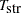be the total number of STRATUM variables

•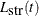be the number of unique values for the tth STRATUM variable, where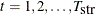• H be the total number of strata

•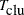be the total number of CLUSTER variables

•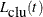be the number of unique values for the tth CLUSTER variable, where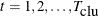•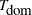be the total number of DOMAIN variables in a domain (you might have multiple domains defined in a DOMAIN statement)

•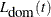be the number of unique values for the tth DOMAIN variable, where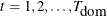• D be the total number of domains

•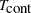be the total number of continuous analysis variables

•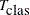be the total number of categorical analysis variables (CLASS variable)

•be the number of unique values for the tth CLASS variable, where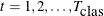•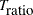be the total number of ratios

•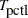be the total number of percentiles

• c be a constant on the order of 32 bytes (64 for 64-bit architectures) plus the maximum combined unformatted and formatted length among all the STRATUM, CLUSTER, DOMAIN, and CLASS variables

If all combinations of levels of categorical variables exist, the maximum potential memory (in bytes) requirements for the analysis is estimated by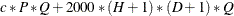where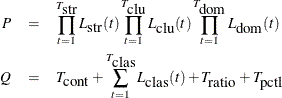A relatively small amount of memory, compared to the memory usage described in the preceding calculation, is also needed for the analysis.

When the data-dependent memory usage overwhelms what is available in the computer system, the procedure might open one or more utility files to complete the analysis. This process can be controlled by the SAS system option SUMSIZE=, which sets the memory threshold where utility file operations begin. For best results, set SUMSIZE= to be less than the amount of real memory that is likely to be available for the task. See the chapter on SAS system options in SAS System Options: Reference for a description of the SUMSIZE= option.

If PROC SURVEYMEANS reports that there is insufficient memory, increase SUMSIZE=. A SUMSIZE= value greater than MEMSIZE= has no effect. Therefore, you might also need to increase MEMSIZE=.

The MEMSIZE option can be specified at system invocation, on the SAS command line, or in a configuration file. However, the MEMSIZE system option is not available in some operating environments. See the SAS Companion for your operating environment for more information and for the syntax specification.

To report a procedure’s memory consumption, you can use the FULLSTIMER option. The syntax is described in the SAS Companion for your operating environment.

Also see the SAS System Options: Reference for more information about how to adjust your computation resource parameters for your operating environment.

For additional information about the memory usage for categorical variables, see the section "Computational Resources" in the chapter "The MEANS Procedure" in the Base SAS Procedures Guide: Statistical Procedures.